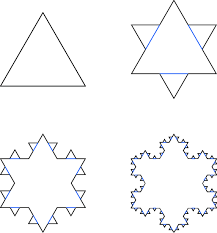A mathematical art form, fractals are infinite geometric constructs that display similar patterns throughout regardless of magnitude. The most popular example, and perhaps easiest to visualise, is the well-known (in the fractal-world) Koch snowflake. This fractal curve is produced by starting with an equilateral triangle and then by following the infinite pattern of adding an equilateral triangle to each side of the shape. The beautiful snowflake design slowly appears.As the name suggests patterns like this are naturally occurring. Snowflakes are a clear example, but they can also be found in clouds, river networks, cauliflowers and systems of blood vessels in the human body!

The snowflake example is particularly interesting mathematically. As the diagram above demonstrates, the first iteration has 3x4 sides, the second 3x42, the third 3x43 as with each step every side of the previous shape is split into 4. After the 𝑛𝑡ℎ iteration the shape would have 3x4𝑛 sides and therefore when the pattern is continued infinitely, the snowflake will have infinite sides and hence also infinite perimeter. Despite an infinite perimeter, the area of the snowflake remains finite, as there is a circle of some fixed perimeter that would always encompass it.

Aside from being visually appealing, it’s properties like these that make fractals so interesting. The pattern used to create a snowflake can be translated into solutions for real-world applications, such as a TV aerial to optimise the surface area with respect to volume.

It’s no wonder that these patterns have started to be applied to music: there are several ways in which translating the ideas to sound can be interpreted. One of the easiest to conceptualise is taking a short tune and using that as your equilateral triangle in the above example – consider the four-note tune that features heavily in The Hunger Games. As discussed previously, these four notes are recognisable because of the ratio of their relative frequencies. So, if they played over and over in a loop, but in such a way that the frequency of the loops matches the same ratio, it’s possible to produce the exact same tune using just a loop of the original notes (but a lot quicker). Repeating this process infinitely would produce a tune that would be self-similar regardless of the scale – a fractal.

A relatively new field, most of the study into musical fractals is simply to create art and news sounds. As studies develop, assuming they follow a similar trend to visual fractals, it will be interesting to see what applications and problems they solve!